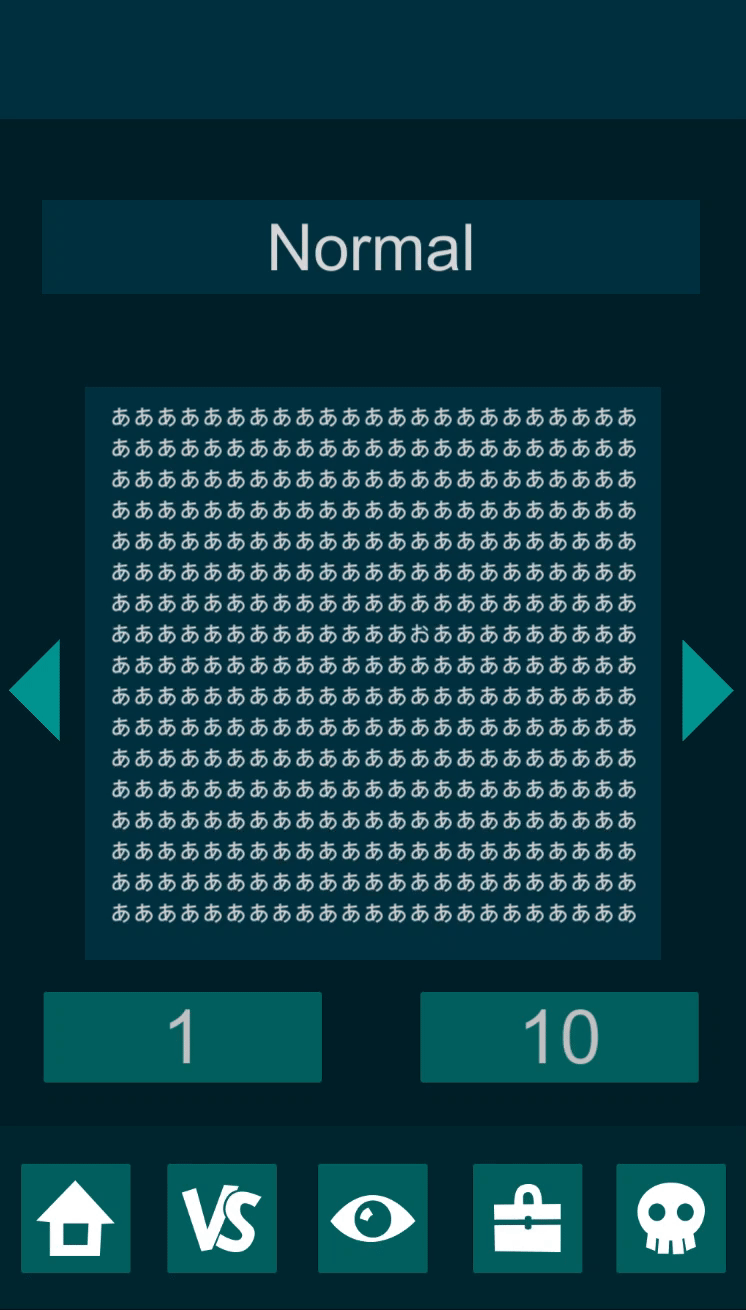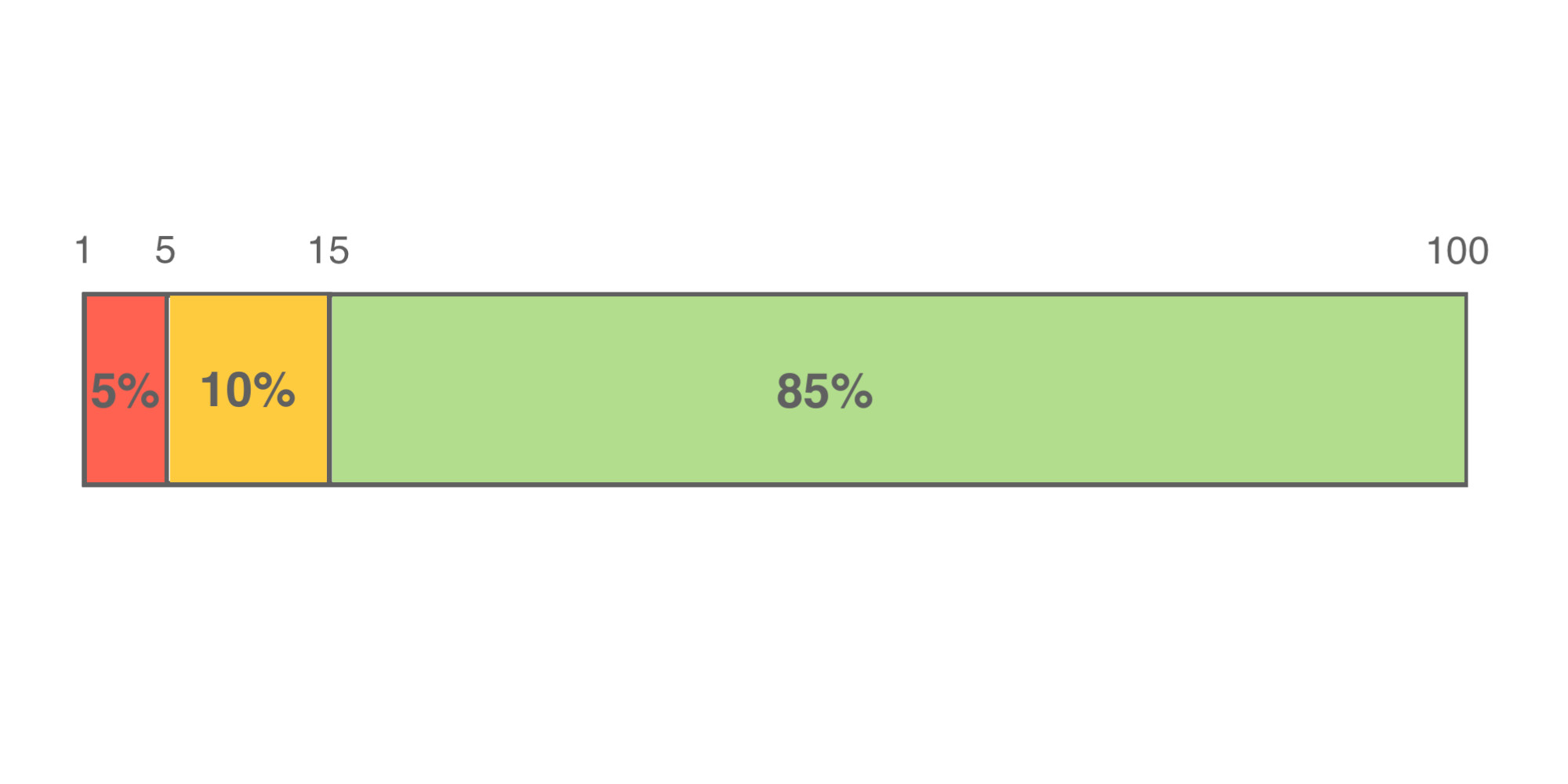Overview

I tried to implement a part of the gacha of the social game.
It has the following simple specifications.
・ Each gacha is a single shot and 10 consecutive.
-Compatible with pickup gacha.
・ Results are displayed in text.

Demo screenClient-side repository.

This is the php code on the backend side.

Gacha.php

<?php

//Lottery of items within the same rarity
function get_item(\$item_rarity,\$gacha_group_id){

//Total gacha weights
\$item_weight_sum = 0;

//Gacha weight array
\$item_weight_array = array();

//Gacha ID array
\$item_id_array = array();

//Create a PDO instance
\$item_pdo = get_PDO();

//Store SELECT statement in variable
\$item_select_sql = "SELECT item_weight,item_id FROM item_config WHERE item_rarity ='". \$item_rarity. "' AND gacha_group_id ='". \$gacha_group_id. "'";

//Execute SQL statement and store result in variable
\$item_select_stmt = \$item_pdo->query(\$item_select_sql);

//Output the contents of the array line by line with the foreach statement
// \$rou['Column name']Can be taken out with
foreach (\$item_select_stmt as \$item_row) {
//Output by database field name
\$item_weight_sum += (int)\$item_row['item_weight'];
\$item_weight_array[] = (int)\$item_row['item_weight'];
\$item_id_array[] = (int)\$item_row['item_id'];
}

//Random number generation
\$random = rand(1,\$item_weight_sum);
\$item_weight_total=0;

//Item ID lottery
foreach(\$item_weight_array as \$item_key => \$item_value ){
\$item_weight_total += \$item_value;
if(\$random <= \$item_weight_total){
\$item_result = \$item_id_array[\$item_key];
break;
}
}

//Extract rarity and item name from item ID
\$item_sql = "SELECT item_rarity,item_name FROM item WHERE item_id ='". \$item_result. "'";
\$item_stmt = \$item_pdo->query(\$item_sql);
foreach(\$item_stmt as \$item){
echo 'Rarity: ' . \$item['item_rarity'] . '　';
echo 'Item name: ' . \$item['item_name'];
echo "\n";

}

}

//Rarity lottery
function get_rarity(\$gacha_group_id){

try{
//Total gacha weights
\$gacha_weight_sum = 0;
//Gacha weight array
\$gacha_weight_array = array();
//Gacha Rarity Array
\$gacha_rarity_array = array();

//Extracted based on gacha group
\$dbh = get_PDO();
\$sql = "SELECT gacha_weight,gacha_rarity FROM rarity_config WHERE gacha_group_id ='". \$gacha_group_id. "'";
\$stmt = \$dbh->query(\$sql);

//Narrow down the conditions in the gacha group and add up the weights of the gacha
foreach (\$stmt as \$row) {
//Output by database field name
\$sum += (int)\$row['gacha_weight'];
\$gacha_weight_array[] = (int)\$row['gacha_weight'];
\$gacha_rarity_array[] = (int)\$row['gacha_rarity'];
}

//Random number generation
\$random = rand(1,\$sum);
\$gacha_weight_total=0;

//Lottery logic(Use random numbers to find weights that were within range)
foreach(\$gacha_weight_array as \$key => \$value ){
\$gacha_weight_total += \$value;
if(\$random <= \$gacha_weight_total){
//Weights that were within range
\$rarity_result = \$gacha_rarity_array[\$key];
break;
}
}

//Item lottery
get_item(\$rarity_result,\$gacha_group_id);

//Describe the processing when an error (exception) occurs
}catch (PDOException \$e) {

//Display an error message
echo 'Unable to access the database!' . \$e->getMessage();

//forced termination
exit;
}
}

//PDO instantiation
function get_PDO(){
return new PDO(
'mysql:host=mysql1.php.starfree.ne.jp;dbname=opnvtw_stdb;charset=utf8',
'opnvtw_lantitor',
'1211stst',
[PDO::ATTR_ERRMODE => PDO::ERRMODE_EXCEPTION,
PDO::ATTR_DEFAULT_FETCH_MODE => PDO::FETCH_ASSOC,
]
);
}

//First function called
function get_gacha(){

//Describe PDO processing in try
try {

\$gacha_id = \$_REQUEST['gacha_id'];

\$pdo = get_PDO();
\$stmt = \$pdo-> prepare("SELECT * FROM gacha WHERE gacha_id = ?");
\$stmt->bindValue(1,\$gacha_id,PDO::PARAM_STR);
\$stmt->execute();

//gacha table gacha_Turn the gacha for times.
foreach (\$stmt as \$row) {
for(\$i = 1; \$i <= (int)\$row['gacha_times']; \$i++){
//Rarity lottery, then item lottery
get_rarity(\$row['gacha_group_id']);
}

}
//Describe the processing when an error (exception) occurs
} catch (PDOException \$e) {

//Display an error message
echo 'Unable to access the database!' . \$e->getMessage();

//forced termination
exit;

}

}

get_gacha();

?>

Development environment

MacOS Catalina 10.15.5
Client is C # + Unity 2019.2.12f1
Backend is PHP
Free PHP + MySQL plan for rental server StarSeverFree
This time it is implemented on a rental server, but if you want to check locally, use Xampp.

Back end

Lottery logic

The lottery logic uses a weighted random algorithm.
In the example figure, ☆ 5 is 5%, ☆ 4 is 10%, and ☆ 3 is 85%.
The search range is 1 to 5 for ☆ 5, 6 to 15 for ☆ 4, and 16 to 100 for ☆ 3.
Random values between 1 and 100 are given, and if the value is 60, it is between 16 and 100, so the lottery result will be ☆ 3. The cumulative sum is used for the search.
Using this logic, we are doing a lottery of rarity → lottery of items within the same rarity.GachaLogic.php

//Gacha weight array
\$gacha_weight_array = array(5,10,85);
//Gacha Rarity Array
\$gacha_rarity_array = array(5,4,3);
// 1~Generate random numbers between 100
\$random = rand(1,100);
\$gacha_weight_total=0;

//Find a range of random numbers
foreach(\$gacha_weight_array as \$key => \$value ){
\$gacha_weight_total += \$value;
//Numbers in range
if(\$random <= \$gacha_weight_total){
//Extract the rarity from the rarity array
\$result = \$gacha_rarity_array[\$key];
break;
}
}

echo \$result;

Master data / DB

It is the data of two types of gacha (normal gacha single shot and 10 series, pickup gacha single shot and 10 series).
This time, I would like to explain the overall flow by taking when 10 pickup gachas are pressed as an example.

The Gacha table is the table that stores the details of the gacha.
When the pickup gacha is pressed, the gacha_id pickup_sort_10 is passed to the server side (PHP).
gacha_id is for identifying gacha and is unique.
Since gacha_times 10 means to spin the gacha 10 times, the lottery will be held 10 times.
gacha_group_id can identify the type of gacha.
The number of times and the cost are different between single shot and 10 consecutive shots, but the type is the same.
Therefore, the gacha_group_id of pickup-sort_1 and pickup-sort_10 are both B.

Gacha table

| gacha_id | gacha_group_id | gacha_name | gacha_cost | gacha_times |
|:-:|:-:|:-:|:-:|:-:|
| normal_1 | A | test_1 | 10 | 1 |
| normal_10 | A | test_10 | 100 | 10 |
| pickup_sort_1 | B | test_pickup_sort_1 | 10 | 1 |
| pickup_sort_10 | B | test_pickup_sort_1 | 100 | 10 |

The rareity setting table is a table that sets the weight for each gacha_group_id.
The lottery will be done using the gacha_weight of B, which is the gacha_group_id of the pickup gacha.
There is an 87% chance of getting a ☆ 3, a 10% chance of getting a ☆ 4, and a 3% chance of getting a ☆ 5.
If the total gacha_weight is 1000, it can be in 0.1% increments, and if it is 100, it can be in 1% increments.
Using the gacha_rarity from the lottery here, we will draw the item next.

Rarity setting table

|gacha_group_id|gacha_rarity|gacha_weight|
|:-:|:-:|:-:|
|A|3|870|
|A|4|100|
|A|5|30|
|B|3|870|
|B|4|100|
|B|5|30|

The item setting table is a table that sets the weights for each gacha_group_id and item_rarity.
A lottery will be held using B of gacha_group_id and the rarity (gacha_rarity) that was drawn earlier.
** It takes a lot of time to insert data into the table, but by allowing you to set each one, you can implement the pickup without changing the code. ** **
For example, if gacha_group_id is B and item_id is 4001 or 5001, it is easy to win the lottery even within the same rarity.

Item setting table

|gacha_group_id|item_id|item_rarity|item_weight|
|:-:|:-:|:-:|:-:|
|A|3001|3|100|
|A|3002|3|100|
|A|3003|3|100|
|A|3004|3|100|
|A|3005|3|100|
|A|3006|3|100|
|A|3007|3|100|
|A|3008|3|100|
|A|3009|3|100|
|A|3010|3|100|
|A|4001|4|500|
|A|4002|4|125|
|A|4003|4|125|
|A|4004|4|125|
|A|4005|4|125|
|A|5001|5|333|
|A|5002|5|333|
|A|5003|5|334|
|B|3001|3|100|
|B|3002|3|100|
|B|3003|3|100|
|B|3004|3|100|
|B|3005|3|100|
|B|3006|3|100|
|B|3007|3|100|
|B|3008|3|100|
|B|3009|3|100|
|B|3010|3|100|
|B|4001|4|500|
|B|4002|4|125|
|B|4003|4|125|
|B|4004|4|125|
|B|4005|4|125|
|B|5001|5|500|
|B|5002|5|250|
|B|5003|5|250|

The item table is the table that contains the item details.
You can display the name (item_name) and item type (item_type) using the item_id that was drawn by lottery.

Item table

|item_id|item_rarity|item_name|item_type|
|:-:|:-:|:-:|:-:|
|3001|3|Bubble sort|1|
|3002|3|Heapsort|1|
|3003|3|Linear search|2|
|3004|3|Ray tracing|3|
|3005|3|Euclidean algorithm|3|
|3006|3|Bucket sort|1|
|3007|3|Shellsort|1|
|3008|3|Insertion sort|1|
|3009|3|Selection sort|1|
|3010|3|Shaker sort|1|
|4001|4|Merge sort|1|
|4002|4|Binary search|2|
|4003|4|Dijkstra’s algorithm|3|
|4004|4|Bellman-Ford method|3|
|4005|4|Floyd-Warshall Floyd method|3|
|5001|5|Quick sort|1|
|5003|5|Depth-first search|2|

The above is the implementation on the backend side.

client

The client side is written in Unity, C #.
The UI architecture is implemented with a simple MV (R) P.
I posted a story on Qiita when I implemented it with MV (R) P before, so I will share it.
[Unity] MV (R) P pattern was adopted in the out-game design [Design]
Also, since this time we focused on the back-end implementation and ideas, I will omit the explanation of the client-side implementation.

Finally

In order to actually implement gacha, I think that more detailed table settings and logic will be required.
I have posted some other articles on Qiita, so please have a look.

Tags:

Updated: